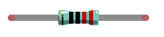# Two LEDs Blink with Arduino

## In this post, we'll see how to Blink an external two LEDs connected digital pins of an Arduino Uno board and how to program itNow, try to make two LEDs blink trough Arduino UNO board.

### Component listArduino UNO x1 Buy NowBreadboard x1 Buy NowUSB cable x1 Buy NowLED x2 Buy NowResistor 220Ω x2 Buy NowJumper M/M x3 Buy Now

## Code knowledge

In the last Lesson, we have a simple understanding of programming. Now let's learn more about basic programming knowledge.

### Parameters of function

In the last chapter, we have used a function with a parameter, such as:

``delay(1000);              // wait for a second``

Next, we will define a function with a parameter as below:

``````void functionA (int i) {
i = i + 1;
}``````

"i" is the parameter of this function. "int" is the type of i. When calling this function, it is necessary to enter the parameter of int type:

``functionA (1);``

The input parameter will be assigned to "i" and involved in the calculation of the functionA(int i):A function can define more than one parameter and the type of the parameters can be different:

``````void functionB (int i, char j) {
char k = 'a';
i = i + 1;
k = j;
}``````

### Boolean data type

Date of Boolean type can only be assigned to "true" or "false".

"true" generally represents a certain relationship which is tenable and correct, and "false" is the opposite.

``````boolean isTrue;
isTrue = true;            // after the execution, "isTrue" is assigned to true.
isTrue = false;           // after the execution, "isTrue" is assigned to false.
)​``````

In the code, the number 0 can be considered to be false, and nonzero numbers can be considered ture.

### Logical operator

The logic operators have "&&" (and), "||" (or), "!" (non), and the calculation object of them are boolean type. The result of logic operation is as follows:For example：

``````boolean isTrue;
isTrue = true && false;   // after the execution, "isTrue"is assigned to false.
isTrue = true || false;      // after the execution, "isTrue"is assigned to true.
isTrue = !true;                // after the execution, "isTrue"is assigned to false.``````

### Relation operator

The relational operator is used to judge whether the relationship of the two amounts is tenable and correct. If the relationship is tenable, the result is true. Otherwise, the result is false.

For example, the results of "1>2" is true and the result of "1<2" is false:

``````boolean isTrue;
isTrue = 1 < 2;           // after the execution, "isTrue"is true.
isTrue = 1 > 2;           // after the execution, "isTrue"is false.``````

There are other relational operators such as "==" (equal to), ">=" (greater than or equal to), "<=" (less than

or equal to) and "=!" (not equal to).

### Conditional statement

Conditional statements are used to decide whether or not to execute the program based on the result of the judgment statement.When there are many statements needed to be executed, we can put them into "{}":Only the section of code in which conditions are tenable will be executed:In addition, it can judge multiple conditions.## Circuit

Arduino  D4 and D5 pin of Arduino UNO to drive these two LEDs respectivelyHardware connection

## Sketch

In order to show the difference between the use of function and no use of the function, we will write two different sketches to make two LEDs blink.

Sketch 2.1.1

At first, use sketch without function to make two LEDs blink alternatively.

``````// set pin numbers:
int led1Pin = 4;            // the number of the LED1 pin
int led2Pin = 5;            // the number of the LED2 pin

void setup() {
// initialize the LED pin as an output:
pinMode(led1Pin, OUTPUT);
pinMode(led2Pin, OUTPUT);
}

void loop() {
digitalWrite(led1Pin, HIGH);   // turn the LED1 on
digitalWrite(led2Pin, LOW);    // turn the LED2 off
delay(1000);                           // wait for a second

digitalWrite(led1Pin, LOW);    // turn the LED1 off
digitalWrite(led2Pin, HIGH);   // turn the LED2 on
delay(1000);                           // wait for a second
}

``````

This section of code is similar with the last section. The difference is the amount of LEDs are two. And two LED blink alternatively.

 Variable scope In the 2,3 rows of code above, we define two variables to store the pin number. These two variables defined outside of the function are called "Global variable", which can be called by all other functions. Variables defined within a function is called "local variable", which can be called only by the current function. When local variables and global variables have same names, the variable names represents local variable in the current function.

Verify and upload the code, then you will see the two LEDs blink alternatively.1000000